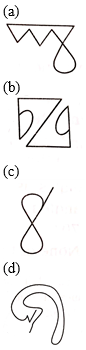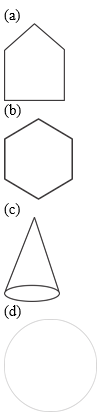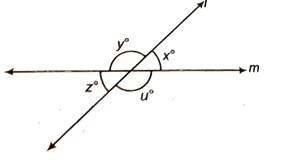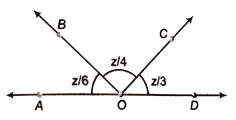### CTET/ UPTET Exam Practice Mathematics Questions

Dear Students!!! There is most general as well as a scoring section in all the competitive entrance examinations in the teaching field i.e "Mathematics".Because in this section only one thing is work i.e your accuracy and that could be nourished with the daily practice.With proper system, Study Notes, Quizzes, Vocabulary one can quiet his/her nerves and exceed expectations in the blink of an eye. So, for this, we are providing you the daily quiz for all teaching exams i.e CTET Exam 2019DSSSB ,KVS,STET Exam.

Q1. Which of the following is an open curve shape?Q3. Which of the following is a shape of three dimensional shape?Q4. The largest chord of a circle is
(a) Semi-circle
(b) Perimeter of circle
(c) Diameter of circle
(d) None of the above(a) 15°
(b) 40°
(c) 25°
(d) 30°(a) 95° and 85°
(b) 108° and 72°
(c) 130° and 50°
(d) 105° and 75°

Q7. Lines L and m intersect at O, forming angles as shown in figure. If x=45°, then respective values of y, z and u are(a) 45°, 135°, 135°
(b) 135°, 135°, 45°
(c) 135°, 45°, 135°
(d) 115°, 45°, 115°

Q8. The value of z (in degrees) in the given figure is(a) 180°
(b) 216°
(c) 240°
(d) 40°(a) 130°
(b) 85°
(c) 110°
(d) 95°

Q10. Find the value of ‘a’(a) 120°
(b) 140°
(c) 90°
(d) 150°

Solutions

S1. Ans.(d)
Sol. Open curve shape is a shape in which both ends are not closed.
Hence, option (d) is correct.

S2. Ans.(c)
Sol. In a given pattern, number are arranged in the following way
14×2=28, 15×2=30, 31×2=62
In option (c) the numbers are arranged in similar way.
02×2=04, 15×2=30, 26×2=52

S3. Ans.(c)
Conical shape is a three-dimensional shape.

S4. Ans.(c)
Sol. The largest chord of circle is the diameter of a circle.